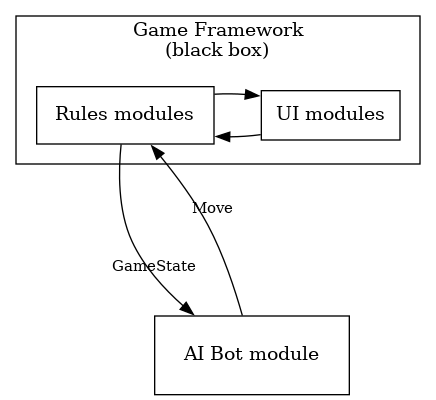# How to move a node in dot "to the right"

Consider the following (simplified) diagram:

``````digraph app_structure {
node [shape=rectangle
margin=0.2]
edge [fontsize=11
minlen=3
]

subgraph cluster_0 {
margin=15
label = <Game Framework<br/>(black box)>
rank = min
node [shape=rectangle
margin=0.2]
edge [minlen=1]
{
rank=same

ui [label=<UI modules>
margin=0.15]
app [label=<Rules modules>]
}
ui -> app
app -> ui
}

bot [label=<AI Bot module>
margin=0.3
]
app -> bot [label="               "
labeldistance=4]
labeldistance=5]
}
``````

This gives with `dot`:

Here I would like to move only the “AI Bot” node half to the right so that it centres with the “Game Framework” cluster. I tried adding some nodes with various rank settings (thinking I could make them invisible later) but somehow could not get there.

Any ideas how to do this?

One way is to add a second set of (invisible) edges between ui and bot, like so:

``````    // slide "bot" to the right by adding symmetric (invisible) edges
edge [style=invis]
ui->bot
bot->ui
``````

Giving:1 Like

So cool. I was playing with nodes, but didn’t think of “nudging” the graph with edges. Thanks heaps!

Now it looks as I want it. Cheers to Graphviz!(However, the ways to tell Graphviz how to move things visually around feel rather cumbersome and limited to me.)